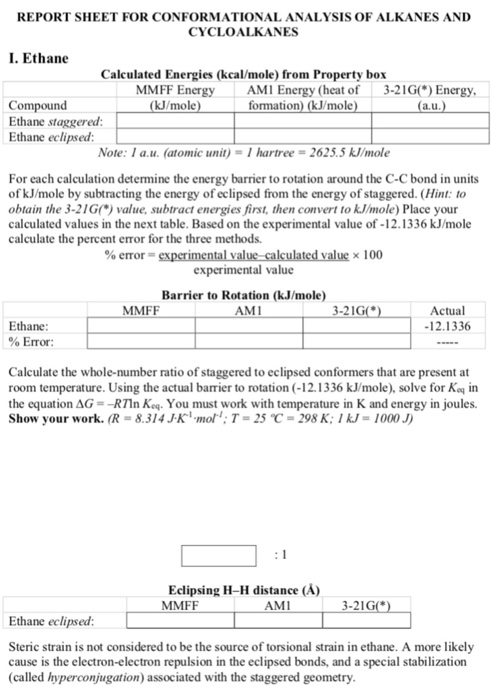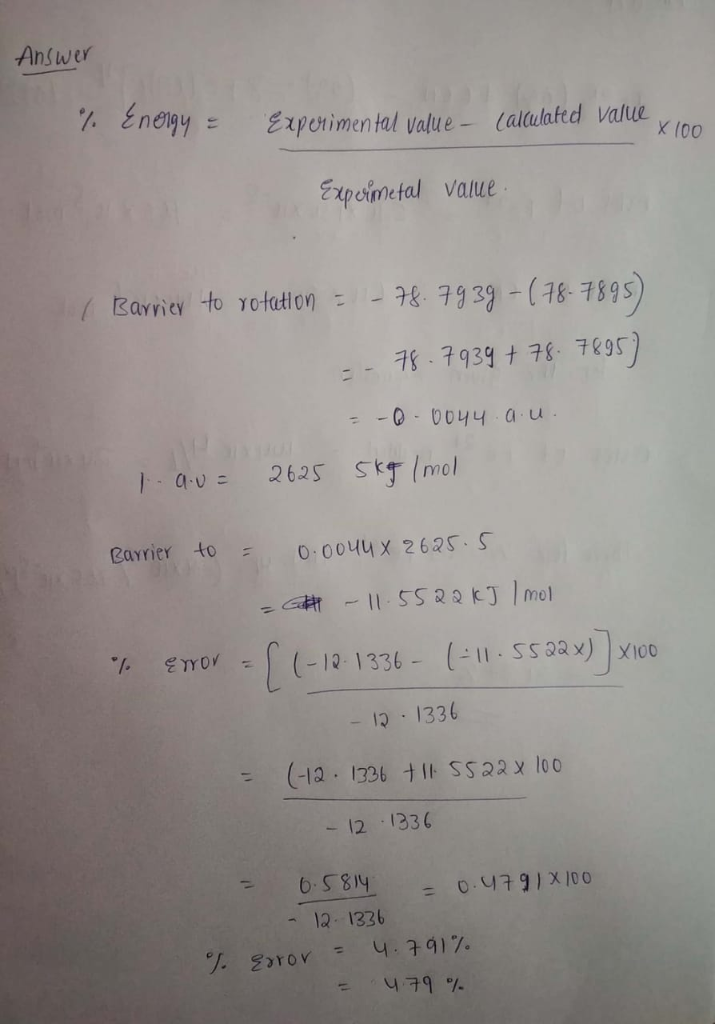# REPORT SHEET FOR CONFORMATIONAL ANALYSIS OF ALKANES AND CYCLOALKANES Ethane caMMFF Calculated Energies (kcal/mole) from Property...REPORT SHEET FOR CONFORMATIONAL ANALYSIS OF ALKANES AND CYCLOALKANES Ethane caMMFF Calculated Energies (kcal/mole) from Property box MMFF Energy AMI Energy (heat of 3-21GO Energy kJ/mole Compound Ethane staggered Ethane eclipsed: formation) (kJ/mole a.u Note: I a.u. (atomic unit)-I hartree 2625.5 kJ/mole For each calculation determine the energy barrier to rotation around the C-C bond in units of kJ/mole by subtracting the energy of eclipsed from the energy of staggered. (Hint: to obtain the 3-21G) value, subtract energies first, then convert to kJ/mole) Place your calculated values in the next table. Based on the experimental value of-12.1336 kJ/mole calculate the percent error for the three methods. %error- x 100 experimental value Barrier to Rotation (kJ/mole) AMI MMF Actual 12.1336 Ethane % Error: Calculate the whole-number ratio of staggered to eclipsed conformers that are present at room temperature. Using the actual barrier to rotation (-12.1336 k/mole), solve for Koq in the equation ΔG =-R Tin Kol. You must work with temperature in K and energy in joules. Show your work. (R-8.314JK mol T- 25 C-298 K:1kJ-1000J) Eclipsing H-H distance (A) MMFF AMI Ethane eclipsed: Steric strain is not considered to be the source of torsional strain in ethane. A more likely cause is the electron-electron repulsion in the eclipsed bonds, and a special stabilization (called hyperconjugation) associated with the staggered geometry#### Earn Coin

Coins can be redeemed for fabulous gifts.